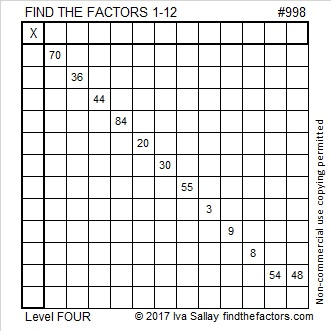# 998 and Level 4

I could easily turn this level 4 puzzle into a level 3 puzzle by rearranging the clues so that you would know an ideal order to work on the clues. Since I’m not doing that, you will have to think more to solve this or any other level 4 puzzle. It still won’t be that hard to do. You will just have to look at all the clues and think about which one should be used next. Remember to always use logic when you consider each clue. Guessing and checking will only frustrate you!Print the puzzles or type the solution in this excel file: 12 factors 993-1001

Here’s a little bit about the number 998:

998 is palindrome 828 in BASE 11 because 8(121) + 2(11) + 8(1) = 998

• 998 is a composite number.
• Prime factorization: 998 = 2 × 499
• The exponents in the prime factorization are 1 and 1. Adding one to each and multiplying we get (1 + 1)(1 + 1) = 2 × 2 = 4. Therefore 998 has exactly 4 factors.
• Factors of 998: 1, 2, 499, 998
• Factor pairs: 998 = 1 × 998 or 2 × 499
• 998 has no square factors that allow its square root to be simplified. √998 ≈ 31.5911This site uses Akismet to reduce spam. Learn how your comment data is processed.